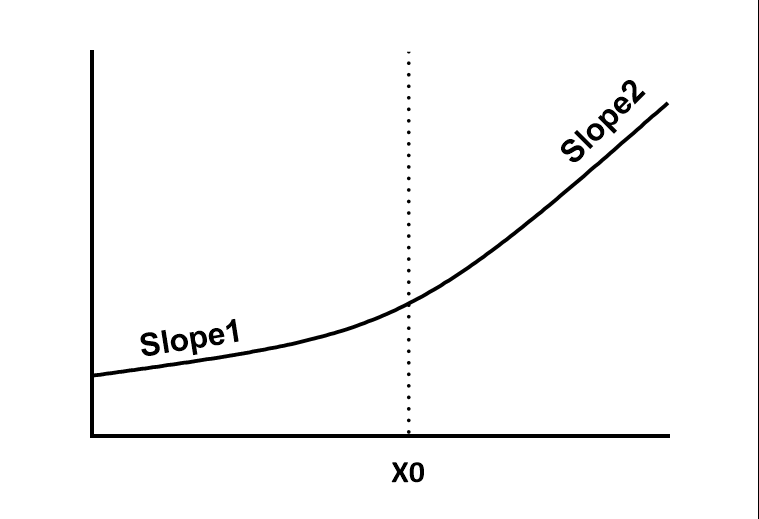## Please enable JavaScript to view this site.

 Equation: Hinge function. Segmental regression lines with gentle connection

## Introduction

This hinge function fits one line to all data points with X less than some value X0, and another line to all points with X greater than X0, while ensuring that the two lines intersect at X0. The difference between this and segmental linear regression is that there is a gentle curve connecting the two lines. This hinge function was derived by Andrew Gelman.

## Caution

Don't use hinge function  to analyze a biphasic Scatchard or Lineweaver-Burk plot. A biphasic Scatchard plot follows a curve, not two intersecting lines. There is no abrupt break point. You should fit the original data to a two-site binding curve instead.

## Step by step

Create an XY data table. Enter time into X, and your measurements into Y. If you have several experimental conditions, place the first into column A, the second into column B, etc.

After entering data, click Analyze, choose nonlinear regression, choose the panel equations for lines, and choose Hinge function.

Consider if you want to constrain X0 (usually you will) or Delta to constant values.

## Model

Y= Intercept + Slope1*(X – X0) + (Slope2 – Slope1)*Delta*ln(1+exp((X-X0)/Delta))

## Interpret the parameters

Intercept is the Y value X=0

Slope1 is the slope of the first line segment, expressed in Y units divided by X units.

Slope2 is the slope of the second line segment, expressed in Y units divided by X units.

X0 is the X value where the two line segments would intersect, if there were no curve connecting them. Often you will want to constrain this to a constant value equal to the time you applied an experimental intervention.

Delta defines how sharp the bend is. When delta is close to zero, the result is is very close to two intersecting lines (segmental regression). As Delta is made larger, the curve joining the two lines is more spread out. Delta must always be positive. You may want to set this to a constant and then try various values. Unless there are lots of data near the inflection, it may not work to ask Prism to fit it.